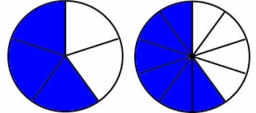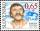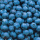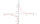# Equivalent fractions

Are these two fractions equivalent -4/9 and 11/15?

Result

x=

### Step-by-step explanation:Did you find an error or inaccuracy? Feel free to write us. Thank you!

Showing 1 comment:John2010
GreatTips to related online calculators
Need help to calculate sum, simplify or multiply fractions? Try our fraction calculator.

## Related math problems and questions:

• Equivalent fractions 2Write the equivalent multiplication expression. 2 1/6÷3/4
• Land costThe land is worth 33295 CZK. 1st owner has 5/15, 2nd owner has 4/15, 3rd owner has 1/5 4th owner has 1/5. How much CZK each of them will receive?
• Simplest form of a fractionWhich one of the following fraction after reducing in simplest form is not equal to 3/2? a) 15/20 b) 12/8 c) 27/18 d) 6/4
• CompareCompare fractions (34)/(3) and (12)/(4). Which fraction of the lower?
• Comparing and sortingArrange in descending order this fractions: 2/7, 7/10 & 1/2
• SallySally is making 15 individual blueberry cheesecakes. How many pounds of blueberries will be in each cheesecake if 5 1/4 pounds of blueberries are divided equally among them?
• To improper fractionChange mixed number to improper fraction a) 1 2/15 b) -2 15/17
• 15 numberWhat number is smaller (greater) by 15 than its half?
• How many 15How many six-eights are there in 18? Which of the following would you choose to solve this question? 18 x 6/8 or 18 : 6/8
• ReciprocalsWhich among the given reciprocal is correct a. 3/15x1/3= 1 b. 3/20x20/3=1 c. 7/14x7/7=1 d. 34/3x34/34=1
• Equivalent expressionPablo rewrites the expression 8y-24 as 8(y-3). Hana rewrites 8y-24 as 2(4y-12). Are Pablo’s and Hana’s expressions each equivalent to 8y-24? Explain your reasoning.
• ExpressionIf it is true that (l + 15 w)/(w) = 6, then the value of the expression (13 l)/(12 w) is:
• Sort fractionsWhich is larger 3/7, 3/8, 3/9, 3/6 =
• Multiplication negativesJosh says that (4)(-7)(-1) is equal to (4)(7). Evangelina says (4)(-7)(-1) is equal to (-28)(-1) . Who is correct? Explain your reasoning.
• Paper boxCalculate whether 11 dm² of paper is sufficient for gluing a box without a lid with bottom dimensions of 2 dm and 15 cm and 12 cm high. Write result as: 0 = No, 1 = Yes
• Giraffes to monkeysThe ratio of the number of giraffes to the number of monkeys in a zoo is 2 to 5. Which statement about the giraffes and monkeys could be true? A. For every 10 monkeys in the zoo, there are 4 giraffes. B. For every  giraffe in the zoo, there are 3 monkeys.
• The sum 12The sum of 3 mixed numbers is 20 13/15. two of the numbers are 6 1/3 and 7 5/6. what is the third number?